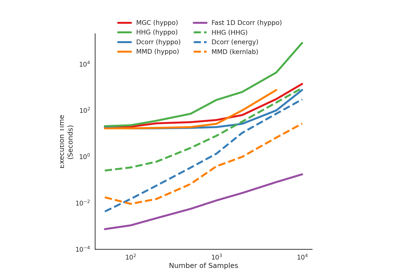HHG¶

class hyppo.independence.HHG(compute_distance='euclidean', **kwargs)

Heller Heller Gorfine (HHG) test statistic and p-value.

This is a powerful test for independence based on calculating pairwise Euclidean distances and associations between these distance matrices. The test statistic is a function of ranks of these distances, and is consistent against similar tests 1. It can also operate on multiple dimensions 1.

Parameters
• compute_distance (str, callable, or None, default: "euclidean") -- A function that computes the distance among the samples within each data matrix. Valid strings for compute_distance are, as defined in sklearn.metrics.pairwise_distances,

• From scikit-learn: ["euclidean", "cityblock", "cosine", "l1", "l2", "manhattan"] See the documentation for scipy.spatial.distance for details on these metrics.

• From scipy.spatial.distance: ["braycurtis", "canberra", "chebyshev", "correlation", "dice", "hamming", "jaccard", "kulsinski", "mahalanobis", "minkowski", "rogerstanimoto", "russellrao", "seuclidean", "sokalmichener", "sokalsneath", "sqeuclidean", "yule"] See the documentation for scipy.spatial.distance for details on these metrics.

Set to None or "precomputed" if x and y are already distance matrices. To call a custom function, either create the distance matrix before-hand or create a function of the form metric(x, **kwargs) where x is the data matrix for which pairwise distances are calculated and **kwargs are extra arguements to send to your custom function.

• **kwargs -- Arbitrary keyword arguments for compute_distance.

Notes

The statistic can be derived as follows 1:

Let $$x$$ and $$y$$ be $$(n, p)$$ samples of random variables $$X$$ and $$Y$$. For every sample $$j \neq i$$, calculate the pairwise distances in $$x$$ and $$y$$ and denote this as $$d_x(x_i, x_j)$$ and $$d_y(y_i, y_j)$$. The indicator function is denoted as $$\mathbb{1} \{ \cdot \}$$. The cross-classification between these two random variables can be calculated as

$A_{11} = \sum_{k=1, k \neq i,j}^n \mathbb{1} \{ d_x(x_i, x_k) \leq d_x(x_i, x_j) \} \mathbb{1} \{ d_y(y_i, y_k) \leq d_y(y_i, y_j) \}$

and $$A_{12}$$, $$A_{21}$$, and $$A_{22}$$ are defined similarly. This is organized within the following table:

 $$d_x(x_i, \cdot) \leq d_x(x_i, x_j)$$ $$d_x(x_i, \cdot) \leq d_x(x_i, x_j)$$ $$d_x(x_i, \cdot) \leq d_x(x_i, x_j)$$ $$A_{11} (i,j)$$ $$A_{12} (i,j)$$ $$A_{1 \cdot} (i,j)$$ $$d_x(x_i, \cdot) > d_x(x_i, x_j)$$ $$A_{21} (i,j)$$ $$A_{22} (i,j)$$ $$A_{2 \cdot} (i,j)$$ $$A_{\cdot 1} (i,j)$$ $$A_{\cdot 2} (i,j)$$ $$n - 2$$

Here, $$A_{\cdot 1}$$ and $$A_{\cdot 2}$$ are the column sums, $$A_{1 \cdot}$$ and $$A_{2 \cdot}$$ are the row sums, and $$n - 2$$ is the number of degrees of freedom. From this table, we can calculate the Pearson's chi squared test statistic using,

$S(i, j) = \frac{(n-2) (A_{12} A_{21} - A_{11} A_{22})^2} {A_{1 \cdot} A_{2 \cdot} A_{\cdot 1} A_{\cdot 2}}$

and the HHG test statistic is then,

$\mathrm{HHG}_n (x, y) = \sum_{i=1}^n \sum_{j=1, j \neq i}^n S(i, j)$

The p-value returned is calculated using a permutation test using hyppo.tools.perm_test.

References

1(1,2,3)

Ruth Heller, Yair Heller, and Malka Gorfine. A consistent multivariate test of association based on ranks of distances. Biometrika, 100(2):503–510, 2013.

Methods Summary

 HHG.statistic(x, y) Helper function that calculates the HHG test statistic. HHG.test(x, y[, reps, workers, random_state]) Calculates the HHG test statistic and p-value.

HHG.statistic(x, y)

Helper function that calculates the HHG test statistic.

Parameters

x,y (ndarray) -- Input data matrices. x and y must have the same number of samples. That is, the shapes must be (n, p) and (n, q) where n is the number of samples and p and q are the number of dimensions. Alternatively, x and y can be distance matrices, where the shapes must both be (n, n).

Returns

stat (float) -- The computed HHG statistic.

HHG.test(x, y, reps=1000, workers=1, random_state=None)

Calculates the HHG test statistic and p-value.

Parameters
• x,y (ndarray) -- Input data matrices. x and y must have the same number of samples. That is, the shapes must be (n, p) and (n, q) where n is the number of samples and p and q are the number of dimensions. Alternatively, x and y can be distance matrices, where the shapes must both be (n, n).

• reps (int, default: 1000) -- The number of replications used to estimate the null distribution when using the permutation test used to calculate the p-value.

• workers (int, default: 1) -- The number of cores to parallelize the p-value computation over. Supply -1 to use all cores available to the Process.

Returns

• stat (float) -- The computed HHG statistic.

• pvalue (float) -- The computed HHG p-value.

Examples

>>> import numpy as np
>>> from hyppo.independence import HHG
>>> x = np.arange(7)
>>> y = x
>>> stat, pvalue = HHG().test(x, y)
>>> '%.1f, %.2f' % (stat, pvalue)
'160.0, 0.00'

In addition, the inputs can be distance matrices. Using this is the, same as before, except the compute_distance parameter must be set to None.

>>> import numpy as np
>>> from hyppo.independence import HHG
>>> x = np.ones((10, 10)) - np.identity(10)
>>> y = 2 * x
>>> hhg = HHG(compute_distance=None)
>>> stat, pvalue = hhg.test(x, y)
>>> '%.1f, %.2f' % (stat, pvalue)
'0.0, 1.00'

Examples using hyppo.independence.HHG¶